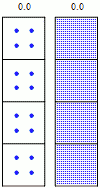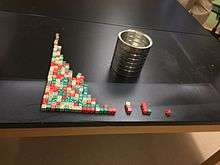# Half-life

Number of
half-lives
elapsed
Fraction
remaining
Percentage
remaining
0 1/1 100
1 1/2 50
2 1/4 25
3 1/8 12.5
4 1/16 6.25
5 1/32 3.125
6 1/64 1.563
7 1/128 0.781
... ... ...
n 1/2n 100/(2n)

Half-life (abbreviated t1⁄2) is the time required for a quantity to reduce to half its initial value. The term is commonly used in nuclear physics to describe how quickly unstable atoms undergo, or how long stable atoms survive, radioactive decay. The term is also used more generally to characterize any type of exponential or non-exponential decay. For example, the medical sciences refer to the biological half-life of drugs and other chemicals in the body. The converse of half-life is doubling time.

The original term, half-life period, dating to Ernest Rutherford's discovery of the principle in 1907, was shortened to half-life in the early 1950s. Rutherford applied the principle of a radioactive element's half-life to studies of age determination of rocks by measuring the decay period of radium to lead-206.

Half-life is constant over the lifetime of an exponentially decaying quantity, and it is a characteristic unit for the exponential decay equation. The accompanying table shows the reduction of a quantity as a function of the number of half-lives elapsed.

## Probabilistic nature of half-lifeSimulation of many identical atoms undergoing radioactive decay, starting with either 4 atoms per box (left) or 400 (right). The number at the top is how many half-lives have elapsed. Note the consequence of the law of large numbers: with more atoms, the overall decay is more regular and more predictable.

A half-life usually describes the decay of discrete entities, such as radioactive atoms. In that case, it does not work to use the definition that states "half-life is the time required for exactly half of the entities to decay". For example, if there are 3 radioactive atoms with a half-life of one second, there will not be "1.5 atoms" left after one second.

Instead, the half-life is defined in terms of probability: "Half-life is the time required for exactly half of the entities to decay on average". In other words, the probability of a radioactive atom decaying within its half-life is 50%.

For example, the image on the right is a simulation of many identical atoms undergoing radioactive decay. Note that after one half-life there are not exactly one-half of the atoms remaining, only approximately, because of the random variation in the process. Nevertheless, when there are many identical atoms decaying (right boxes), the law of large numbers suggests that it is a very good approximation to say that half of the atoms remain after one half-life.

There are various simple exercises that demonstrate probabilistic decay, for example involving flipping coins or running a statistical computer program.

## Formulas for half-life in exponential decay

Main article: Exponential decay

An exponential decay can be described by any of the following three equivalent formulas:where

• N0 is the initial quantity of the substance that will decay (this quantity may be measured in grams, moles, number of atoms, etc.),
• N(t) is the quantity that still remains and has not yet decayed after a time t,
• t1⁄2 is the half-life of the decaying quantity,
• τ is a positive number called the mean lifetime of the decaying quantity,
• λ is a positive number called the decay constant of the decaying quantity.

The three parameters t1⁄2, τ, and λ are all directly related in the following way:where ln(2) is the natural logarithm of 2 (approximately 0.693).

By plugging in and manipulating these relationships, we get all of the following equivalent descriptions of exponential decay, in terms of the half-life:Regardless of how it's written, we can plug into the formula to get

•as expected (this is the definition of "initial quantity")
•as expected (this is the definition of half-life)
•; i.e., amount approaches zero as t approaches infinity as expected (the longer we wait, the less remains).

### Decay by two or more processes

Some quantities decay by two exponential-decay processes simultaneously. In this case, the actual half-life T1⁄2 can be related to the half-lives t1 and t2 that the quantity would have if each of the decay processes acted in isolation:For three or more processes, the analogous formula is:For a proof of these formulas, see Exponential decay § Decay by two or more processes.

### ExamplesHalf life demonstrated using dice in a classroom experiment

There is a half-life describing any exponential-decay process. For example:

• The current flowing through an RC circuit or RL circuit decays with a half-life of RCln(2) or ln(2)L/R, respectively. For this example, the term half time might be used instead of "half life", but they mean the same thing.
• In a first-order chemical reaction, the half-life of the reactant is ln(2)/λ, where λ is the reaction rate constant.
• In radioactive decay, the half-life is the length of time after which there is a 50% chance that an atom will have undergone nuclear decay. It varies depending on the atom type and isotope, and is usually determined experimentally. See List of nuclides.

The half life of a species is the time it takes for the concentration of the substance to fall to half of its initial value.

## Half-life in non-exponential decay

Main article: Rate equation

The decay of many physical quantities is not exponential—for example, the evaporation of water from a puddle, or (often) the chemical reaction of a molecule. In such cases, the half-life is defined the same way as before: as the time elapsed before half of the original quantity has decayed. However, unlike in an exponential decay, the half-life depends on the initial quantity, and the prospective half-life will change over time as the quantity decays.

As an example, the radioactive decay of carbon-14 is exponential with a half-life of 5,730 years. A quantity of carbon-14 will decay to half of its original amount (on average) after 5,730 years, regardless of how big or small the original quantity was. After another 5,730 years, one-quarter of the original will remain. On the other hand, the time it will take a puddle to half-evaporate depends on how deep the puddle is. Perhaps a puddle of a certain size will evaporate down to half its original volume in one day. But on the second day, there is no reason to expect that one-quarter of the puddle will remain; in fact, it will probably be much less than that. This is an example where the half-life reduces as time goes on. (In other non-exponential decays, it can increase instead.)

The decay of a mixture of two or more materials which each decay exponentially, but with different half-lives, is not exponential. Mathematically, the sum of two exponential functions is not a single exponential function. A common example of such a situation is the waste of nuclear power stations, which is a mix of substances with vastly different half-lives. Consider a mixture of a rapidly decaying element A, with a half-life of 1 second, and a slowly decaying element B, with a half-life of 1 year. In a couple of minutes, almost all atoms of element A will have decayed after repeated halving of the initial number of atoms, but very few of the atoms of element B will have done so as only a tiny fraction of its half-life has elapsed. Thus, the mixture taken as a whole will not decay by halves.

## Half-life in biology and pharmacology

Main article: Biological half-life

A biological half-life or elimination half-life is the time it takes for a substance (drug, radioactive nuclide, or other) to lose one-half of its pharmacologic, physiologic, or radiological activity. In a medical context, the half-life may also describe the time that it takes for the concentration of a substance in blood plasma to reach one-half of its steady-state value (the "plasma half-life").

The relationship between the biological and plasma half-lives of a substance can be complex, due to factors including accumulation in tissues, active metabolites, and receptor interactions.

While a radioactive isotope decays almost perfectly according to so-called "first order kinetics" where the rate constant is a fixed number, the elimination of a substance from a living organism usually follows more complex chemical kinetics.

For example, the biological half-life of water in a human being is about 9 to 10 days, though this can be altered by behavior and various other conditions. The biological half-life of cesium in human beings is between one and four months.Look up half-life in Wiktionary, the free dictionary.Wikimedia Commons has media related to Half times.